# Multiplication + variations - math problems

#### Number of problems found: 90

• 2nd class variationsFrom how many elements you can create 2450 variations of the second class?
• VariationsDetermine the number of items when the count of variations of fourth class without repeating is 42 times larger than the count of variations of third class without repetition.
• MedalsIn how many ways can be divided gold, silver and bronze medal among 21 contestant?
• Disco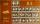On the disco goes 12 boys and 15 girls. In how many ways can we select four dancing couples?
• Subsets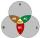How many are all subsets of set ??
• Possible combinations - word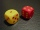How many ways can the letters F, A, I, R be arranged?
• Pins 2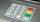how many different possible 4 digits pins can be found on the 10-digit keypad?
• Olympics metalsIn how many ways can be win six athletes medal positions in the Olympics? Metal color matters.
• Combinatorics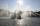The city has 7 fountains. Works only 6. How many options are there that can squirt ?
• Seven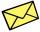Seven friends agree to send everyone a holiday card. How many postcards were sent?
• Tricolors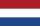From the colors - red, blue, green, black and white, create all possible tricolors.
• Bits, bytes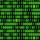Calculate how many different numbers can be encoded in 16-bit binary word?
• Tokens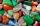In the non-transparent bags are red, white, yellow, blue tokens. We 3times pull one tokens and again returned it, write down all possibilities.
• Six on diceWhat is the probability that when throwing two dice will fall at least one six?
• Vans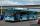In how many ways can 9 shuttle vans line up at the airport?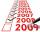Determine the number of integers from 1 to 106 with ending four digits 2015.How many ways can we thread 4 red, 5 blue, and 6 yellow beads onto a thread?
• Word MATEMATIKAHow many words can be created from the phrase MATEMATIKA by changing the letters' order, regardless of whether the words are meaningful?
• Football league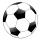In the 5th football league is 10 teams. How many ways can be filled first, second and third place?
• Practice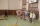How many ways can you place 20 pupils in a row when starting on practice?

Do you have an interesting mathematical word problem that you can't solve it? Submit a math problem, and we can try to solve it.

We will send a solution to your e-mail address. Solved examples are also published here. Please enter the e-mail correctly and check whether you don't have a full mailbox.

Please do not submit problems from current active competitions such as Mathematical Olympiad, correspondence seminars etc...

See also our variations calculator. Multiplication Problems. Variations - math word problems.